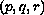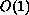Electron. J. Diff. Eqns., Vol. 2002(2002), No. 50, pp. 1-22.

### Metastability in the shadow system for Gierer-Meinhardt's equations Pieter de Groen & Georgi Karadzhov

Abstract:
In this paper we study the stability of the single internal spike solution of the shadow system for the Gierer-Meinhardt equations in one space dimension. It is well-known, that the linearization around this spike consists of a differential operator plus a non-local term. For parameter values in certain subsets of the 3D-parameter space we prove that the non-local term moves the negativeeigenvalue of the differential operator to the positive (stable) half plane and that an exponentially small eigenvalue remains in the negative half plane, indicating a marginal instability (dubbed ``metastability''). We also show, that for parametersin another region, theeigenvalue remains in the negative half plane. In all asymptotic approximations we compute rigorous bounds for the order of the error.

Submitted April 17, 2002. Published June 2, 2002.
Math Subject Classifications: 35B25, 35K60.
Key Words: Spike solution, singular perturbations, reaction-diffusion equations, Gierer-Meinhardt equations

Show me the PDF file (424K), TEX file, and other files for this article.Pieter de Groen Vrije Universiteit Brussel Pleinlaan 2 B-1050 Brussels, Belgium email: pdegroen@vub.ac.be Georgi E. Karadzhov Bulgarian academy of Sciences Institute for Mathematics and Informatics Sofia, Bulgaria email: geremika@math.bas.bg